0
0
01. 云栖社区>
2. 博客>
3. 正文

SVG

Path元素

path元素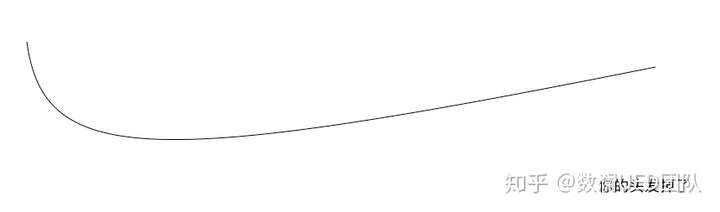OK，这根头发我们已经在屏幕上放好了，如果你将path元素的曲线无限放大会发现，其实它是由非常多的坐标点相互连接组成的。这个时候脑洞放一下，如果我们能获取到这些点是不是就是获取了线的绘制轨迹。就可以逐帧绘制飞线了动效了。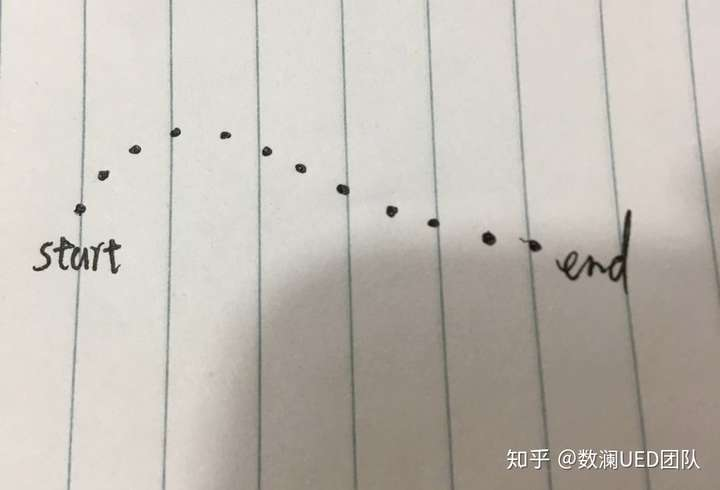SVGPathElement.getPointAtLength

const pointNum = 1500

const pointNum = 1500
const path = d3.select('#line')
const pathline = path.node()
const totalLength = pathline.getTotalLength()
const points = []

const unit = totalLength / pointNum

for (let i = 0; i <= pointNum; i += 1) {
points.push(pathline.getPointAtLength(i * unit))
}

飞线动效-1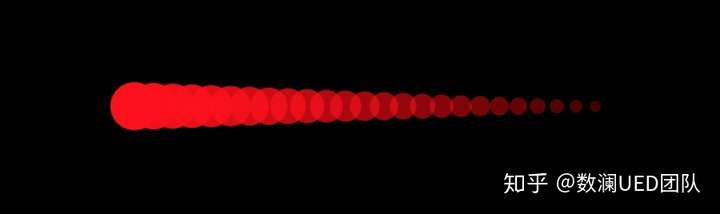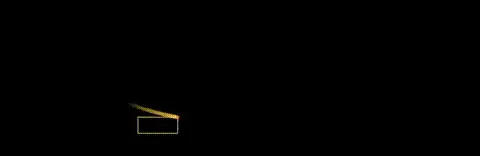class FlyLine {
totalNum = 1500
lineLen = 150
speed = 15
fill = 'rgb(255, 200, 65)'
circles = []
constructor(){
// percent的用处会在后面体现
this.percent = this.lineLen
}
}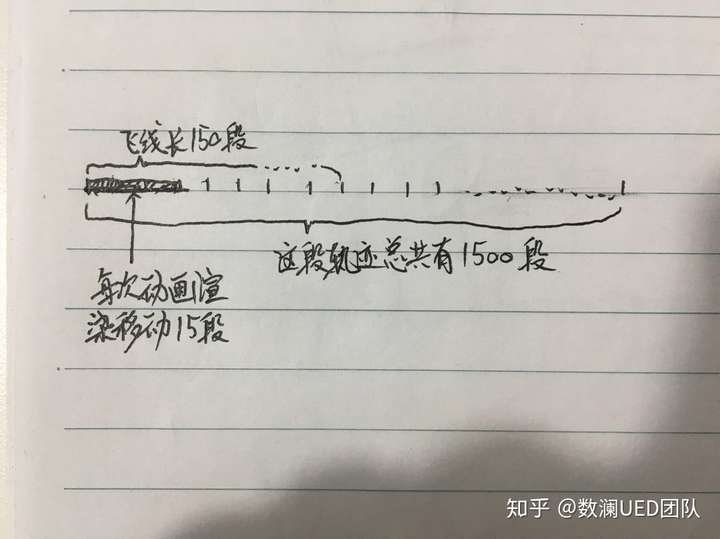class FlyLine {
...
...
_drawCircle(cx, cy, i) {
const {radius, circles, fill} = this
if (circles[i]) {
circles[i].attr("cx", cx).attr("cy", cy)
} else {
circles.push(
circleG1
.append("circle")
.attr("cx", cx)
.attr("cy", cy)
.attr("fill", fill)
.attr('fill-opacity', i * 0.001)
)
}
}
}

class FlyLine {
...
...
_drawFlyLine(){
const {points, percent, lineLen} = this
for (let i = percent - lineLen, j = 0; i < percent; i += 1, j += 1) {
this._drawCircle(points[i].x, points[i].y, j)
}
}
}class FlyLine {
...
...
animate() {
const {lineLen, speed, totalNum} = this
this._drawFlyLine()
this.percent = this.percent + speed > totalNum ? len : this.percent + speed
requestAnimationFrame(() => this.animate())
}
}

FlyLine.animate方法本质上就是个复读机，一遍一遍的让percent变量由小到大变化，控制飞线由起始点到轨迹终点移动。FlyLine._drawFlyLine方法的作用就是根据percent变量的值创建or更新飞线位置。

FlyLine._drawCircle就更不用说了，苦逼小弟，创建or更新circle元素的属性。percent变量更新一次，它要被苦逼的调用lineLen次。

1. 飞线的长度不能超过我们对轨迹分割的段数。

2. 画一根飞线就要生成/更新几百个circle元素，浪费浏览器性能。

const path = container
.append('path')
.attr('fill', 'none')
.attr('stroke', 'none')
.attr('d', 'M50, 50  600, 50')
.attr('id', 'line')

const pathline = path.node()
const len = pathline.getTotalLength()

const animate = () => {
container.select('#flyline').remove()
container.append('path')
.attr('stroke', '#19D0DC')
.attr('fill', 'none')
.attr('id', 'flyline')
.attr('stroke-width', '3px')
.transition()
.duration(5000)
.attrTween('d', function(d) {
const coord = path.attr('d').replace(/(M|Q)/g, '').match(/((\d|\.)+)/g)
var x1 = +coord, y1 = +coord // 起点
return function(t) {
const p = pathline.getPointAtLength(t * len)
return M${x1},${y1} ${p.x},${p.y}
}
})
}

setInterval(animate, 5200)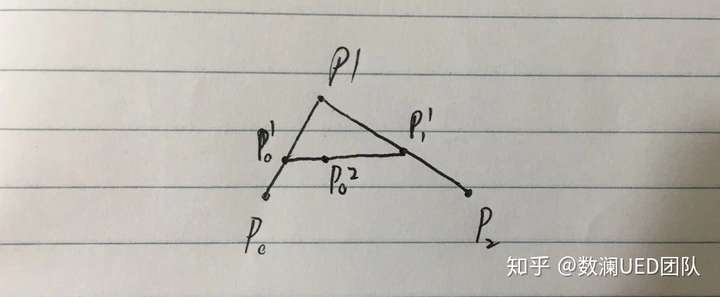.attrTween('d', function(d) {
const coord = path.attr('d').replace(/(M|Q)/g, '').match(/((\d|\.)+)/g)
var x1 = +coord, y1 = +coord, // 起点
x2 = +coord, y2 = +coord, // 控制点
x3 = +coord, y3 = +coord; // 终点
return function(t) {
const p = pathline.getPointAtLength(t * len)
// 根据插值方法进度实时计算当前控制点位置
const x = (1 - t) * x1 + t * x2
const y = (1 - t) * y1 + t * y2
return M${x1},${y1} Q${x},${y} ${p.x},${p.y}
}
})SVG为我们提供了蒙板遮罩等功能，我们只需要在蒙板中定义了一个透明度从内到外逐渐降低径向渐变的圆。然后让他一直跟着飞线的头移动就好了。

const mCircle = d3.select('#m-circle')
.attrTween(function(d){
...
const x = (1 - t) * x1 + t * x2
const y = (1 - t) * y1 + t * y2
mCircle.attr('cx', x)
.attr('cy', y)
})1. 地图与飞线

2. 贝塞尔曲线原理

参考DEMO链接：

https://codepen.io/Narcissus_Li

+ 关注

corcosa 15503人浏览